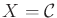# 7. Extensions of Basic Motion Planning

This chapter presents many extensions and variations of the motion planning problem considered in Chapters 3 to 6. Each one of these can be considered as a spin-off'' that is fairly straightforward to describe using the mathematical concepts and algorithms introduced so far. Unlike the previous chapters, there is not much continuity in Chapter 7. Each problem is treated independently; therefore, it is safe to jump to whatever sections in the chapter you find interesting without fear of missing important details.

In many places throughout the chapter, a state spacewill arise. This is consistent with the general planning notation used throughout the book. In Chapter 4, the C-space,, was introduced, which can be considered as a special state space: It encodes the set of transformations that can be applied to a collection of bodies. Hence, Chapters 5 and 6 addressed planning in. The C-space alone is insufficient for many of the problems in this chapter; therefore,will be used because it appears to be more general. For most cases in this chapter, however,is derived from one or more C-spaces. Thus, C-space and state space terminology will be used in combination.

Subsections
Steven M LaValle 2020-08-14